# Chloric acid (HClO3) lewis structure, acid or base, molecular geometry, conjugate base

Home  > Chemistry Article > HClO3 lewis structure and its molecular geometry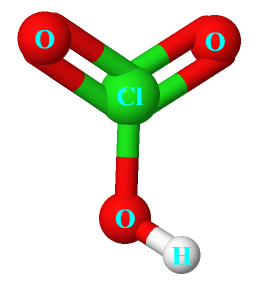Chloric acid is a colorless solution with the chemical formula HClO3. It is also called oxoacid of chlorine. It is a powerful oxidizing agent.

In this tutorial, we will discuss Chloric acid (HClO3) lewis structure, molecular geometry, acid or base, its conjugate base, etc.

Chloric acid can increase the burning of combustible material. It is used for making various chemicals in industries.

 Name of Molecule Chloric acid Chemical formula HClO3 Molecular geometry of HClO3 Trigonal pyramidal Nature Strong acid Total Valence electron for HClO3 26
Page Contents

## How to draw lewis structure of HClO3?

HClO3 lewis structure is made up of one hydrogen, one chlorine, and three oxygen. The chlorine atom is situated in the central position of the HClO3 lewis structure since it is the least electronegative atom.

The central atom of the HClO3 lewis structure violates the octet rule as it contains more than 8 electrons around it.

## Follow some steps for drawing the Lewis structure for HClO3

1. Count total valence electron in HClO3

First of all, determine the valence electron that is available for drawing the lewis structure of HClO3 because the lewis diagram is all about the representation of valence electrons on atoms.

So, an easy way to find the valence electron of atoms in the HClO3 molecule is, just to look at the periodic group of hydrogen, oxygen, and chlorine atoms.

As hydrogen atom belongs to 1st group in the periodic table, chlorine is situated in the 17th group, and oxygen is in the group 16th, hence, the valence electron for hydrogen is 1, for oxygen, it is 6 and for chlorine atom, it is 7.

⇒ Total number of the valence electrons in hydrogen = 1

⇒ Total number of the valence electrons in chlorine = 7

⇒ Total number of the valence electrons in oxygen = 6

∴ Total number of valence electron available for the HClO3 Lewis structure = 1 + 7 + 6×3 = 26 valence electrons         [∴HClO3 molecule has one hydrogen, one chlorine, and three oxygen atoms]2. Find the least electronegative atom and place it at center

An atom with a less electronegative value is more preferable for the central position in the lewis diagram because they are more prone to share the electrons with surrounding atoms.

In the case of the HClO3 molecule, the chlorine atom is less electronegative than the oxygen atom.

So, just put the chlorine atom in the center position and spread three oxygen and one hydrogen atom around it.

Hydrogen is always a terminal or outer atom atom since it can form only one bond.“The hydrogen atoms are bound to the highly electronegative oxygen atom”.

3. Connect outer atoms to central atom with a single bond

In this step, join all outer atoms to the central atom with the help of a single bond.

In, HClO3 molecule, oxygen is the outer atom, and chlorine is the central atom. Hence, joined them. Also, the hydrogen atom will be joined with the oxygen atom.Count the number of valence electrons used in the above structure. There are 4 single bonds used in the above structure, and one single bond means 2 electrons.

Hence, in the above structure, (4 × 2) = 8 valence electrons are used from a total of 26 valence electrons available for drawing the HClO3 Lewis structure.

∴ (26 – 8) = 18 valence electrons

So, we are left with 18 valence electrons more.

4. Place remaining electrons on outer atoms and complete their octet

Let’s start putting the remaining valence electrons on outer atoms first. In the case of the HClO3 molecule, oxygen is the outer atom and it needs 8 electrons in the valence shell to complete the octet.

The hydrogen atom is an exception to the octet because it can hold a maximum of two electrons in its valence level.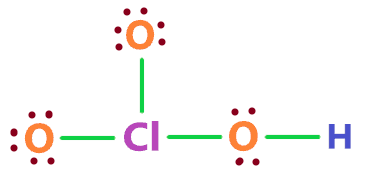So, all oxygen atoms in the above structure completed their octet, because all of them have 8 electrons(electrons represented as dots + 2 electrons in every single bond) in their outermost shell.

Also, the hydrogen atom already completed its octet since it can form only one single bond which contains 2 valence electrons. And in the above figure, the hydrogen atom is attached to the oxygen atom with a single bond.

Now again count the number of valence electrons used in the above structure.

In the above structure, 16 electrons are represented as dots + 4 single bonds means 8 electrons.

Hence, (8 + 16) = 24 electrons are used in the above structure from a total of 26 valence electrons available for the HClO3 Lewis structure.

Now we are left with only 2 electrons more.

5. Complete central atom octet and make multiple bonds if necessary

Here, we will complete the octet of the central atom which is chlorine in the HClO3 molecule.

We have 2 remaining valence electrons also, so, just put these 2 remaining electrons around the chlorine atom.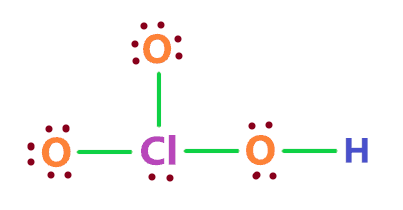In the above figure, the chlorine atom has a total of 8 electrons(three single bonds that contain 6 electrons + 2 electrons as dots), also, we have used all the total valence electrons that are available for drawing the lewis structure of HClO3.

We are done with almost all things, now we have to check the formal charge for the above figure to check its stability.

6. Check the stability with the help of a formal charge concept

The lesser the formal charge on atoms, the better is the stability of the lewis diagram.

To calculate the formal charge on an atom. Use the formula given below-

⇒ Formal charge = (valence electrons – lone pair electrons –  1/2 bonded pair electrons)

Let’s count the formal charge for the 5th step structure.

For chlorine atom

⇒ Valence electrons of chlorine = 7

⇒ Lone pair electrons on chlorine = 2

⇒ Shared pair electrons around chlorine (3 single bonds) = 6

∴ (7 – 2 – 6/2) = +2 formal charge on the chlorine central atom.

You can calculate the formal charge for every atom in HClO3.It is obvious that the above HClO3 lewis structure is not stable because of the high formal charge.

We can reduce the formal charge on the above structure by converting the lone pairs on outer atoms to a covalent bond.

Chlorine atom can hold more than 8 electrons because it has d orbital for extra electrons needed for bonding.

So, just convert the lone pair of oxygen atoms to a covalent bond as shown in the figure below.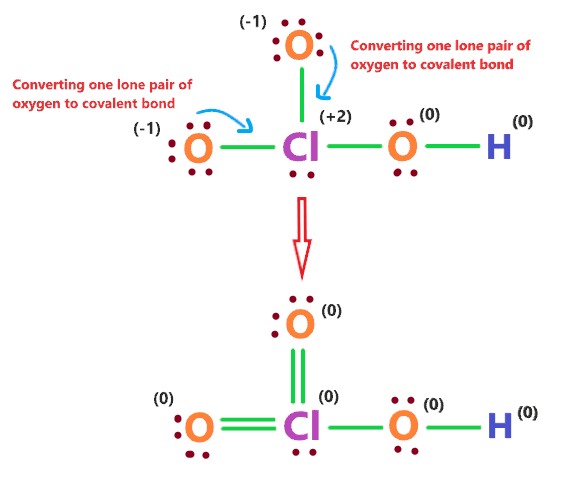We have successfully reduced the formal charge on the HClO3 lewis structure by converting the one lone pair of oxygen atoms to a covalent bond, now each atom gets a formal charge equal to zero.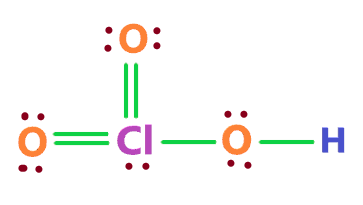### HClO3 Lewis structure

Hence, the above lewis structure of HClO3 is the most stable and appropriate.

## What is the molecular geometry of HClO3?

The molecular geometry of HClO3 is trigonal pyramidal since its central atom chlorine is attached with the three atoms and it also contains one lone pair, which means, it is surrounded by the four regions of electron density that implies, its geometry around chlorine will be pyramidal.

The AXE model for the HClO3 is AX3E1, which correspond to a trigonal pyramidal geometry.HClO3 molecular geometry

## Is HClO3 acid or base? and its Conjugate base

An acid is something that furnishes H+ ions on dissolving in an aqueous solution according to the Arrhenius theory.

Is HClO3 an acid or base? Chloric acid (HClO3) is a strong acid as it is completely ionized in an aqueous solution, no parts of H+ remain bound to it, which means the concentration of hydrogen ion increased in the solution.

⇒ HClO3 → H+ + ClO3

The higher the concentration of H+ ions, the higher is the acidic strength.

Now, what is the conjugate base of HClO3? The conjugate base of HClO3 is a Chlorate ion(ClO3).

The conjugate base is a base that formed when the acid compound loses one proton.

## FAQ

### How many double bonds, single bonds, lone pairs, and bond pairs present in the lewis structure of HClO3?

There are two double bonds, Cl=O and Cl=O, and two single bonds, Cl-O and O-H are present in the lewis structure of HClO3.

Lone pair are those which doesn’t take part in chemical bonding and are represented as dots in the lewis diagram, the bond pairs are referred to as the covalent bonds and represented as a line in the lewis diagram.

So, the bond pairs in the HClO3 lewis diagram are 6 and the lone pair is 7.

### Why does Chlorine in the HClO3 lewis structure violate the octet rule?

Generally, the octet rule said an atom is most stable when it has 8 electrons around it, more than this, we can say the atom violates the octet.

So, in the case of the HClO3 Lewis structure, the chlorine central is attached to two double bonds(Cl=O) and one single bond(Cl-O), also, it contains one lone pair.

We can say the Chlorine atom has 5 bond pairs and 1 lone pair.

Therefore, the total number of electrons in the valence shell of the chlorine atom in the HClO3 Lewis structure will be 12 electrons.

Since it is more than 8 electrons, hence, it violates the octet.

Now Chlorine in the HClO3 lewis structure is able to violate the octet because it has d-orbitals in the third principal energy level, hence, it has an extra orbital(d-orbital) for additional electrons needed for bonding.

Properties of Chloric acid

• It has a molar mass of 84.45914 g mol−1.
• It is a colorless solution.
• It is corrosive to metal and tissue.
• It is an oxoacid of chlorine.
• It is a strong oxidizing agent.

Preparation of Chloric acid

It is usually prepared by two methods, the first is, by heating the hypochlorous acid that will produce one mole of chloric acid and two moles of hydrogen chloride.

⇒ 3HClO → HClO3 + 2HCl

The other method is, by the reaction of sulfuric acid with barium chlorate.

⇒ Ba(ClO3)2 + H2SO4 → 2HClO3 + BaSO4

## Summary

• The total valence electron is available for drawing the HClO3 Lewis structure is 26.
• HClO3 is a strong acid and it completely dissociates into the ions in solution.
• The molecular geometry of HClO3 is trigonal pyramidal.
• In the HClO3 Lewis structure, a total of 7 lone pairs and 6 bonded pairs are present.
• The conjugate base of HClO3 is ClO3.
##### Subscribe to Blog via Email

Join 2 other subscribers

Share it...